# Relative atomic mass and relative formula mass

Whenever you see the words ‘relative’ in chemistry, it means the mass number.

Example

Relative atomic mass = find the mass number

Relative formula mass = find the mass numberThink of all the relatives (relatives), of the bull mastiff (mass number).

Note: if you see the phrase ‘atomic mass’ on its own, it’s incorrect. It should always have the word ‘relative’ in front of it.

### Note for higher tier Chemistry students

Relative atomic mass = find the (average) mass number

Relative atomic mass actually infers that the element has isotopes. To find the relative atomic mass, you will need to work out the average mass of the isotopes.

## Relative atomic mass

The relative atomic mass of an element is equal to its mass number.

### Examples of relative atomic mass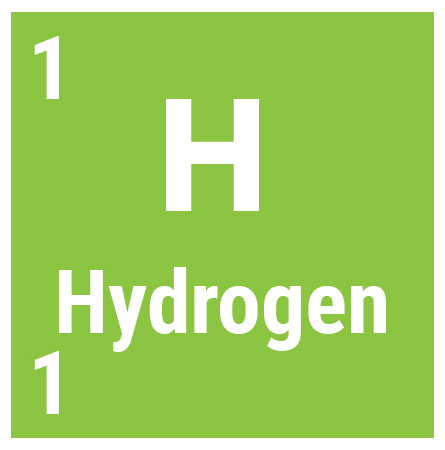Mass number = 1
Atomic number = 1
Relative atomic mass of hydrogen = 1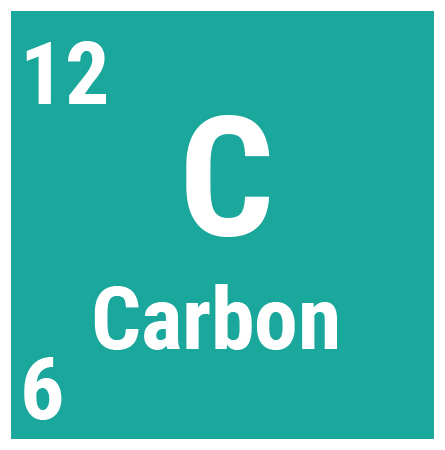Mass number = 12
Atomic number = 6
Relative atomic mass of carbon = 12

## Relative formula mass

To work out the relative formula mass of a compound, just add up all the relative masses within its formula.

### Examples of relative formula mass

#### Water (H2O)Mass number = 1
Atomic number = 1
Relative atomic mass of hydrogen = 1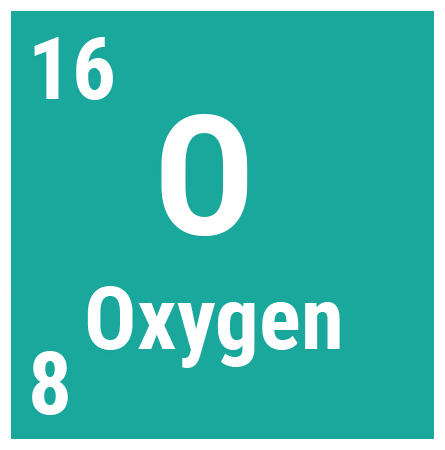Mass number = 16
Atomic number = 8
Relative atomic mass of oxygen = 16

As there are 2 hydrogen atoms and 1 oxygen atom in a water molecule, the calculation is (2x1) + 16 = 18.

Therefore, the relative formula mass of H2O is 18.

#### Sodium chlorideMass number = 23
Atomic number = 11
Relative atomic mass of sodium = 23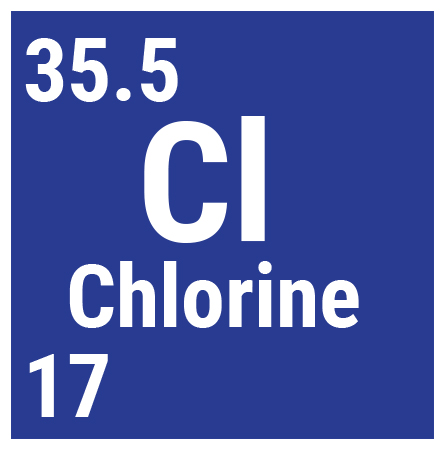Mass number = 35.5
Atomic number = 17
Relative atomic mass of chlorine = 35.5

Sodium chloride molecules consist of a sodium atom and a chlorine atom. The calculation is:

23 + 35.5 = 58.5

So the relative formula mass of NaCl is 58.5

## Finding the relative atomic mass of an element with isotopes

As explained earlier, for most elements the relative atomic mass is equal to their mass number.

However, if you want to find the relative atomic mass of an element with various isotopes, such as chlorine, then you need to find the average atomic mass of its isotopes. You also need to know the relative abundance of the element.

The relative abundance is how much there is of each isotope compared to the total amount of the element.

#### Chlorine

For example, there are two isotopes of chlorine. One has a relative mass of 35 (chlorine-35) and the other has a relative mass of 37 (chlorine-37). In a sample of chlorine gas, 75% is chlorine-35 and 25% is chlorine-37. These percentages are the relative abundances of the isotopes.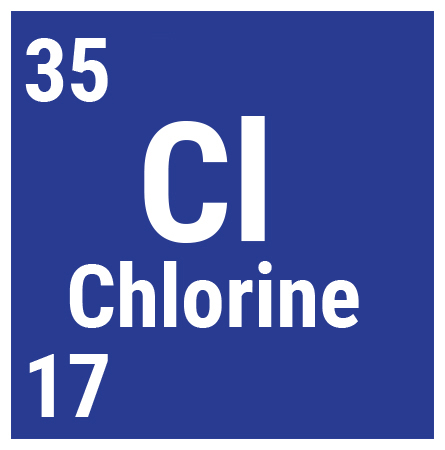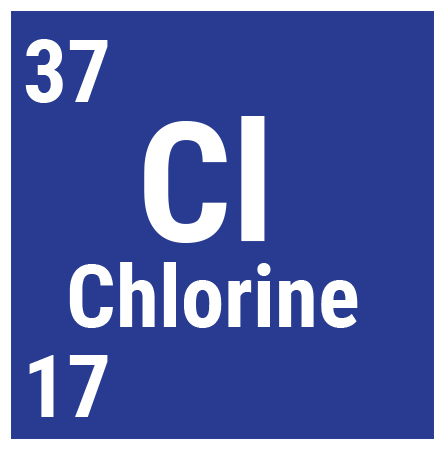To work out relative atomic mass, multiply the mass of each isotope by its relative abundance.  Then add those numbers together and divide by the sum of the relative abundances.

The calculation for working out the relative atomic mass of chlorine is:

(25%x37) + (75%x35)       = 9.25 + 26.25

=35.5

Therefore, the relative atomic mass of chlorine is 35.5.

## Summary

If you see the word ‘relative’, it means mass number.

The relative atomic mass of an element is simply its mass number, unless the element has various isotopes (like chlorine). In this case, find the average of the mass numbers of the isotopes, taking their relative abundances into account.

To work out relative formula mass, add up the mass numbers of all the elements in a compound.